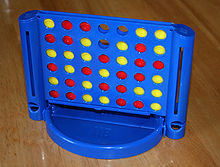KEEL-dataset - data set descriptionThis section describes main characteristics of the connect-4 data set and its attributes:

### General information

Connect-4 data set
TypeClassificationOriginReal world
Features 42(Real / Integer / Nominal)(0 / 0 / 42)
Instances67557 Classes3
Missing values?No

### Attribute description

AttributeDomainAttributeDomainAttributeDomain
A1{x,o,b}A15{x,o,b}A29{x,o,b}
A2{x,o,b}A16{x,o,b}A30{x,o,b}
A3{x,o,b}A17{x,o,b}A31{x,o,b}
A4{x,o,b}A18{x,o,b}A32{x,o,b}
A5{x,o,b}A19{x,o,b}A33{x,o,b}
A6{x,o,b}A20{x,o,b}A34{x,o,b}
A7{x,o,b}A21{x,o,b}A35{x,o,b}
A8{x,o,b}A22{x,o,b}A36{x,o,b}
A9{x,o,b}A23{x,o,b}A37{x,o,b}
A10{x,o,b}A24{x,o,b}A38{x,o,b}
A11{x,o,b}A25{x,o,b}A39{x,o,b}
A12{x,o,b}A26{x,o,b}A40{x,o,b}
A13{x,o,b}A27{x,o,b}A41{x,o,b}
A14{x,o,b}A28{x,o,b}A42{x,o,b}
Class{win,loss,draw}